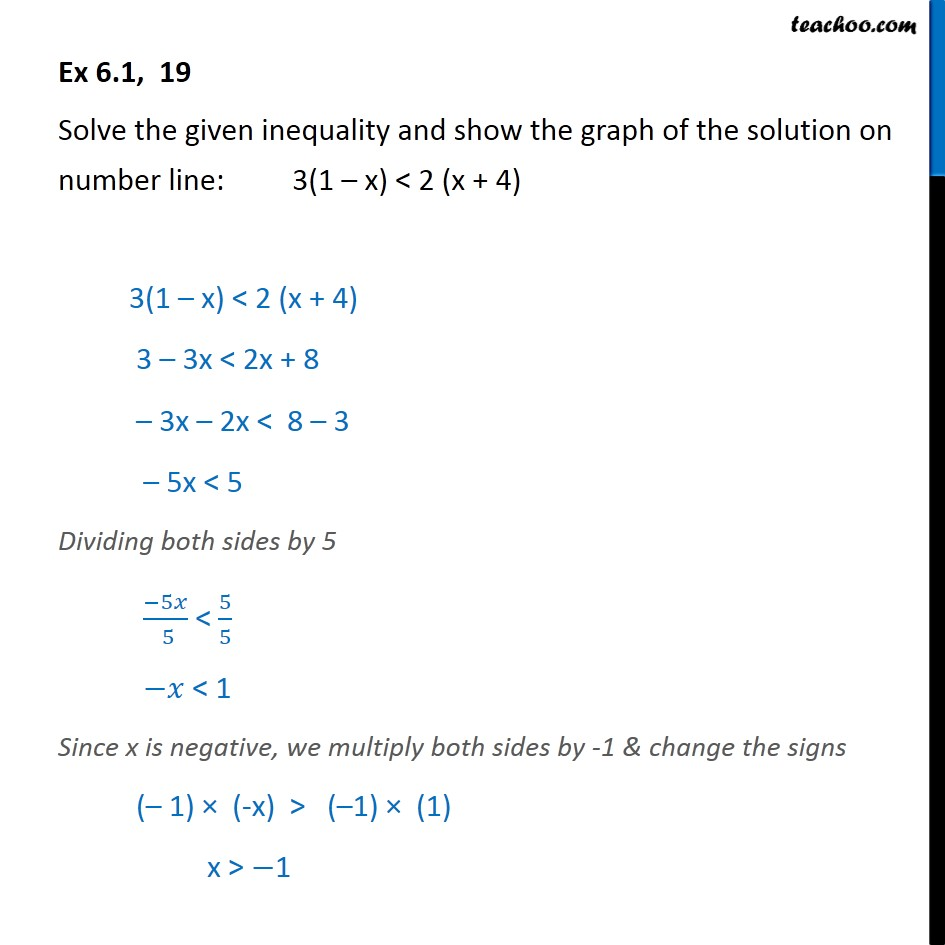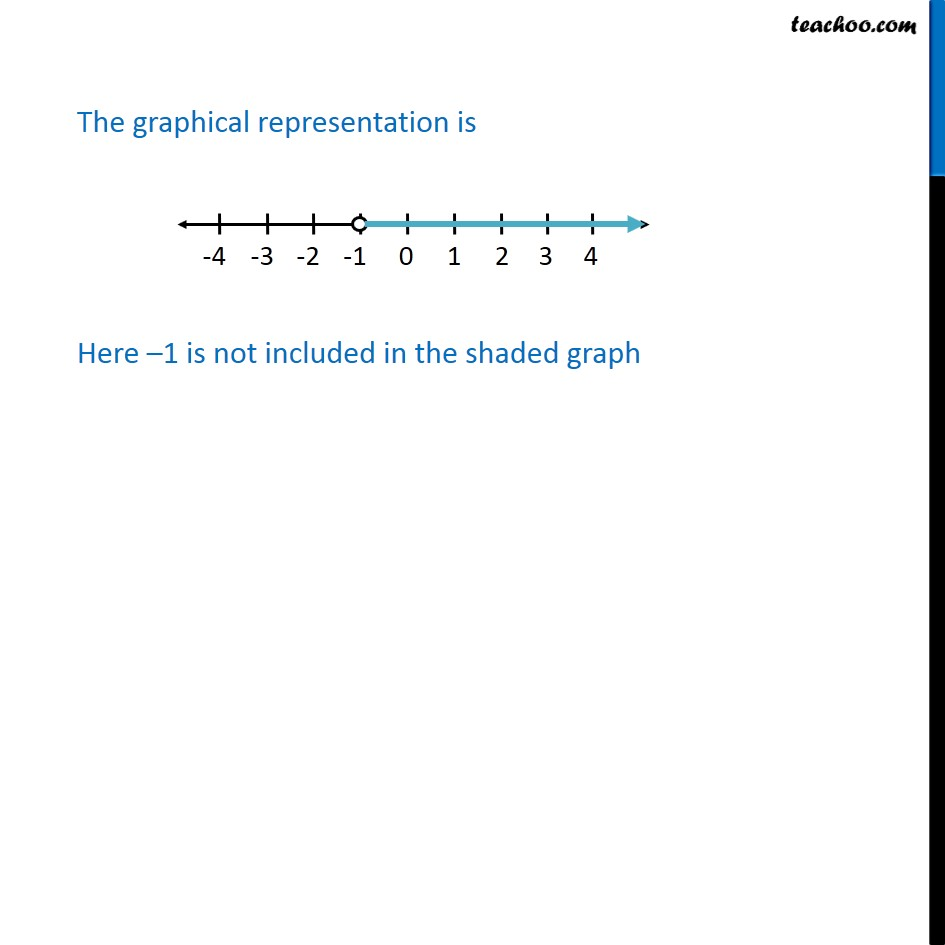Ex 5.1

Chapter 5 Class 11 Linear Inequalities
Serial order wiseLearn in your speed, with individual attention - Teachoo Maths 1-on-1 Class

### Transcript

Ex 5.1, 19 Solve the given inequality and show the graph of the solution on number line: 3(1 – x) < 2 (x + 4) 3(1 – x) < 2 (x + 4) 3 – 3x < 2x + 8 – 3x – 2x < 8 – 3 – 5x < 5 Dividing both sides by 5 ﷐−5𝑥﷮ 5﷯ < ﷐5﷮5﷯ −𝑥 < 1 Since x is negative, we multiply both sides by -1 & change the signs (– 1) × (-x) > (–1) × (1) x > −1 The graphical representation is Here –1 is not included in the shaded graph